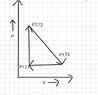# Change in heat and internal energy

Ashshahril
Homework Statement:
What is the change in heat and internal energy in this PV diagram (attached link) from T2 to T3 (T represents temperature)?
Relevant Equations:
ΔQ=mC(T3-T1)
Δu=ΔQ + Δw
ΔQ=mC(T3-T1)

But, will this C be Cp or Cv. Both pressure and volume changes. So, neither of them can be.

Feeling so confused

#### Attachments

•Screenshot_20210622-213430_Chrome.jpg
34.3 KB · Views: 65
•Delta2

vivek_123
Calculate the work from 2-3 which will be the area under the line from 2-3( trapezium area) and you know the change in internal energy= Cv(T2-T3) and u will get ∆Q

•Delta2
Homework Helper
Gold Member
2022 Award
Note this is a full cycle. You start at point 1 and finish at point 1. So the system's final state is the same as its initial state. What can you say about the overall change in the system's internal energy, ΔU?

The area gives you the work done by the gas. ΔW:
If you are dealing with n moles of an ideal gas you can use PV = nRT to get the value of V at each vertex and then derive a formula for ΔW in terms of the P's, T's, n and R.

Then you can find ΔQ.

Make sure the signs (+ or -) of your answers match the sign-convention you are using.

Edit: poor wording corrected.

Last edited:
•Delta2
Mentor
For an ideal gas, internal energy is a function only of temperature. By definition, Cv is given by $$mC_v=\left(\frac{\partial U}{\partial T}\right)_V$$But, since U is dependent only on T for an ideal gas, $$\Delta U=mC_v\Delta T$$

•Delta2